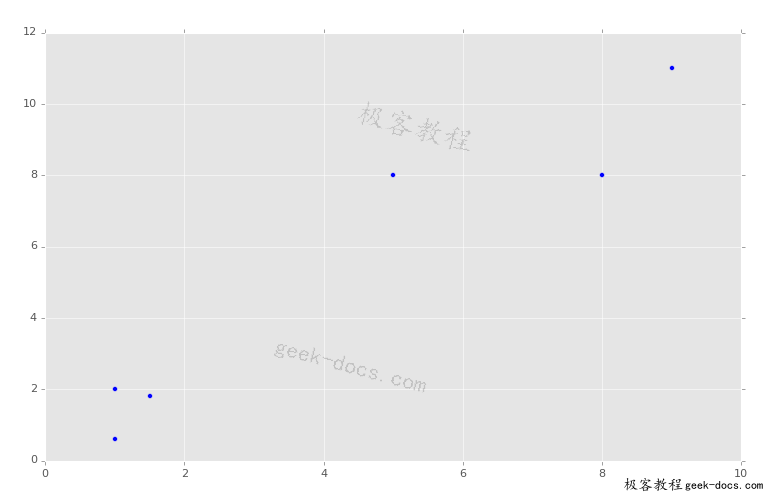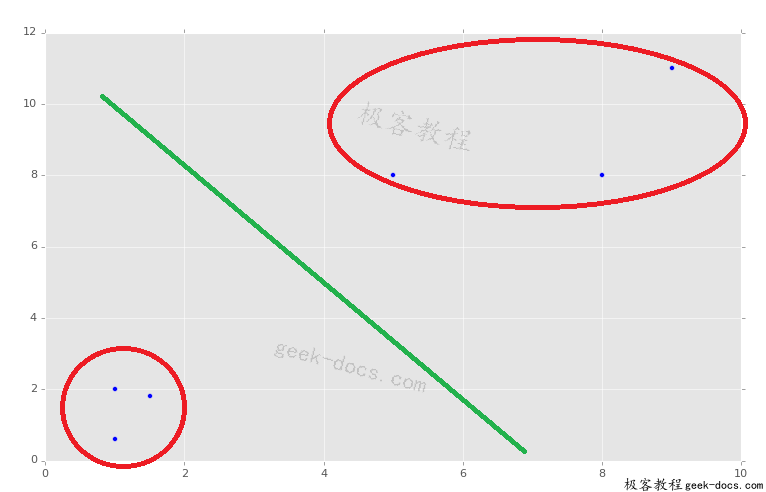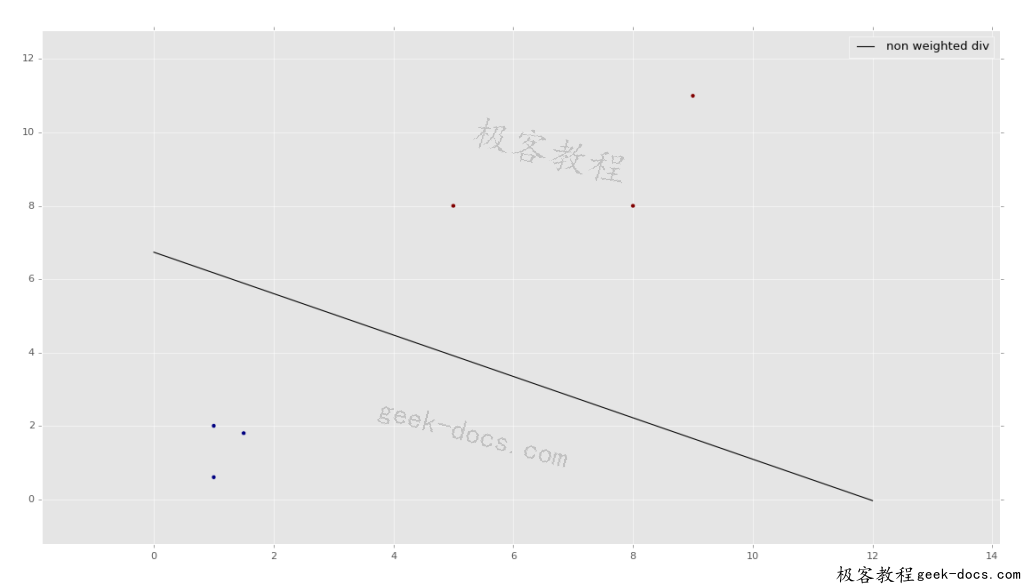# 线性支持向量分类器(SVC)

import numpy as np
import matplotlib.pyplot as plt
from matplotlib import style
style.use("ggplot")
from sklearn import svm


x = [1, 5, 1.5, 8, 1, 9]
y = [2, 8, 1.8, 8, 0.6, 11]

plt.scatter(x,y)
plt.show()X = np.array([[1,2],
[5,8],
[1.5,1.8],
[8,8],
[1,0.6],
[9,11]])

y = [0,1,0,1,0,1]

clf = svm.SVC(kernel='linear', C = 1.0)


clf.fit(X,y)


print(clf.predict([0.58,0.76]))


print(clf.predict([10.58,10.76]))


w = clf.coef_
print(w)

a = -w / w

xx = np.linspace(0,12)
yy = a * xx - clf.intercept_ / w

h0 = plt.plot(xx, yy, 'k-', label="non weighted div")

plt.scatter(X[:, 0], X[:, 1], c = y)
plt.legend()
plt.show()### 评论 1

1. #1

写的很好，但是预测的时候要传2维的
print(clf.predict([0.58,0.76]))
print(clf.predict([[0.58,0.76]]))

Selenium2年前 (2020-07-08)回复

• 回顶